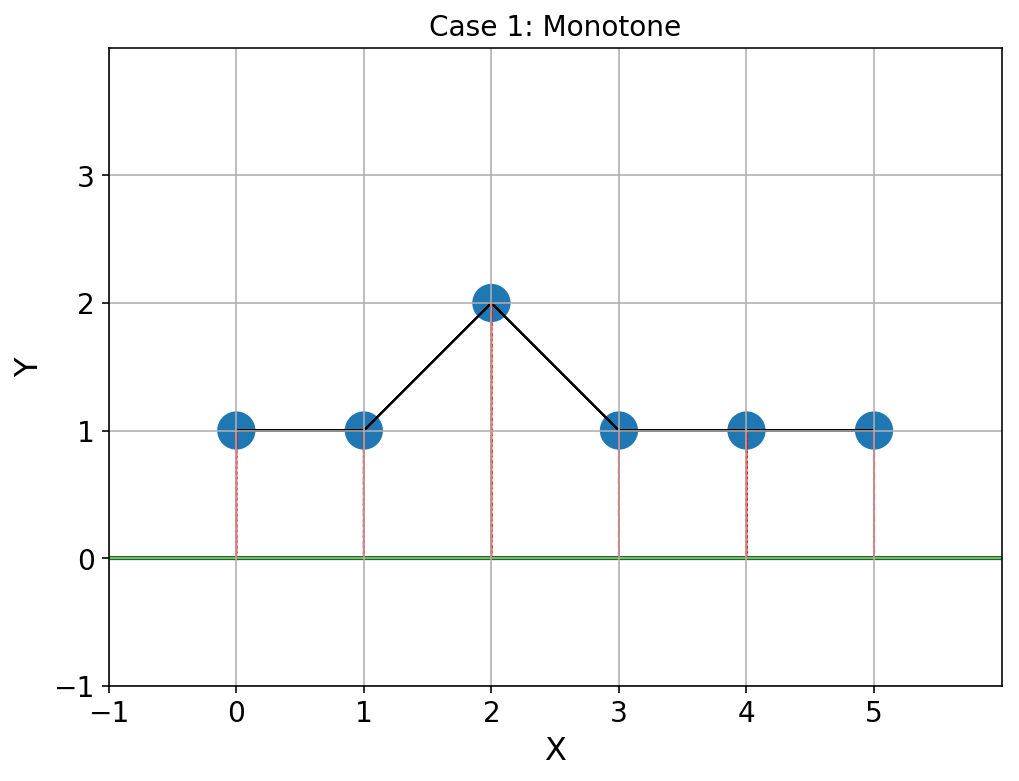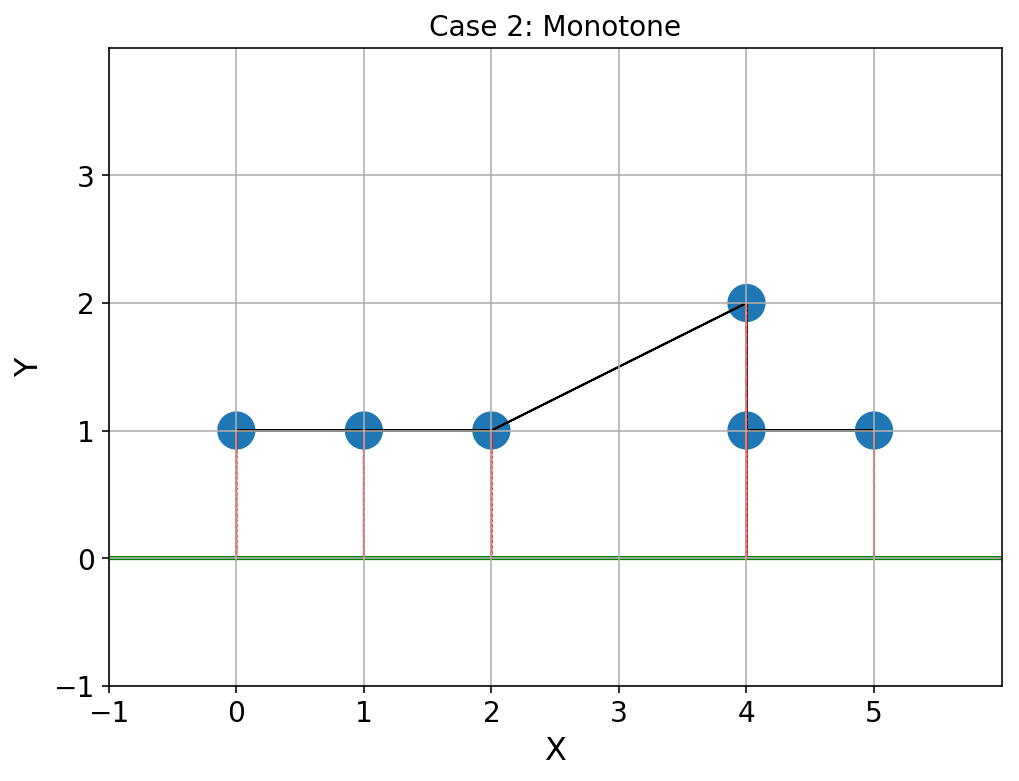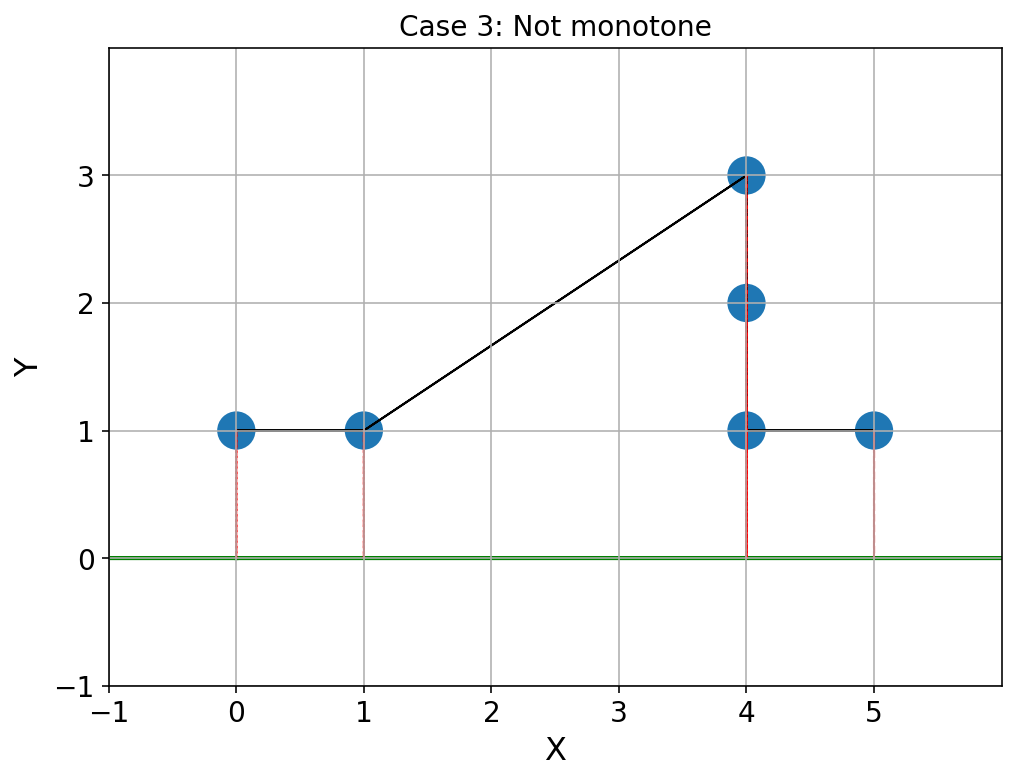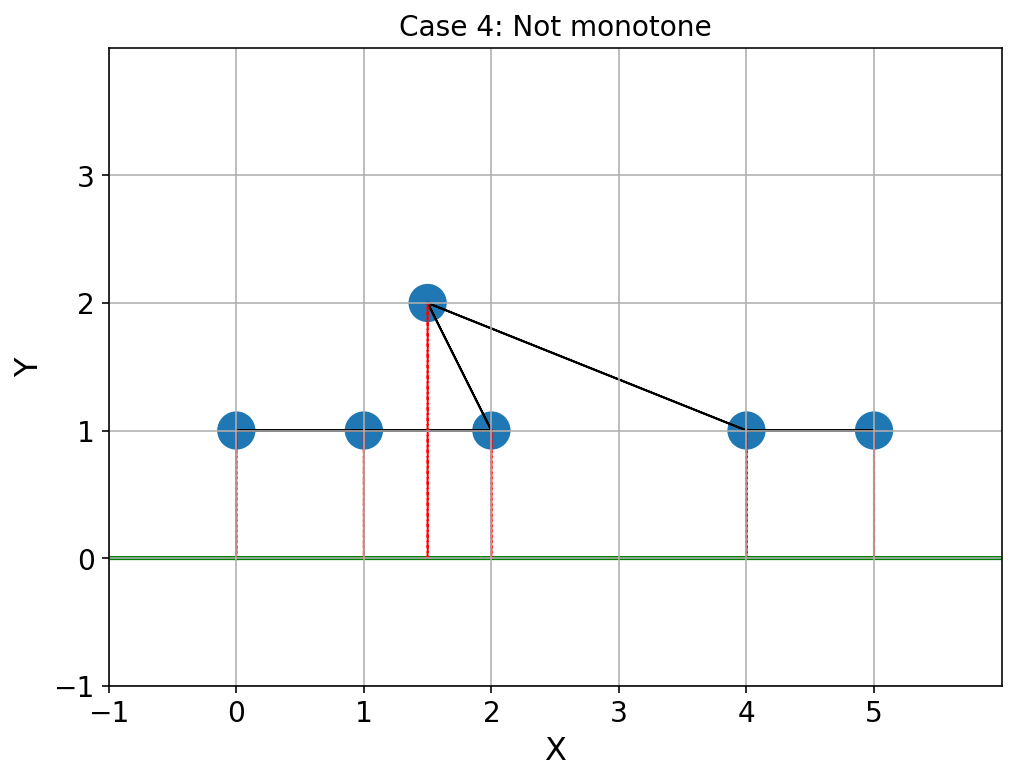In :
from ICGChapter01 import *

In :
# Comment out the line below if the graphics do not display
%config InlineBackend.figure_format = 'retina'

In :
%%javascript
IPython.OutputArea.prototype._should_scroll = function(lines) {return false;}


# Chapter 2: Polygonal chains in two dimensions¶

In this chapter we will explore two dimensional polygonal chains, which are ordered lists of points. Virtually all of the work we do in subsequent chapters will involve polygonal chains in one form or another.

In :
displayTOC("ICGChapter02.ipynb")


# Polygonal chains¶

Contents

• Vertex: the point where two or more lines, line segments or rays meet.

• Polygonal chain: a curve specified by a sequence of points called vertexes, so that the curve consists of the line segments connecting consecutive vertexes. In computer graphics, this is commonly known as a polyline.

• Open polygonal chain: a polygonal chain whose last vertex is not connected to its first vertex.
• Closed polygonal chain: a polygonal chain whose last vertex is connected by a line segment to its first vertex. Alternatively, a polygonal chain whose last and first vertexes are identical.
• Edge: a line segment joining two vertexes.

A polygonal chain $C$ has vertexes $v_1, v_2, ...v_n$, where $n \geq 3$.

We can represent polygonal chains as PointLists. Here is a trivial function to check if a PointList is a polygonal chain:

In :
def isPolygonalChain(plist):
"""If there are three or more points in a list of Points then it is a polygonal chain."""
return len(plist) >= 3


If the chain is open (the default), it has edges $e_1, e_2, ...e_{n-1}$. These edges are the line segments $(v_1, v_2), (v_2, v_3)...(v_{n-1}, v_n)$. Note that when we say "polygonal chain", we will mean "open polygonal chain" unless we state otherwise.

If the chain is closed, it has edges $e_1, e_2, ...e_{n}$. These edges are the line segments $(v_1, v_2), (v_2, v_3)...(v_{n-1}, v_n), (v_n, v_1)$. In the closed chain the last vertex is connected to the first. A closed polygonal chain forms a polygon.

We first of all write a function, edgeIndexes(...) that calculates the indexes of the edges and then we use this to get the edges themselves. Generally speaking, functions that work with indexes rather than directly with the vertexes are easier to understand, develop and test.

In :
def edgeIndexes(n):
"""Given n points in sequence, return the index pairs (i,j) for each edge."""
return [[i, i+1] for i in range(n-1)]

def edges(plist):
"""Given a list of Point objects, return a list of the edges as pairs of Points."""
return [[plist[e], plist[e]] for e in edgeIndexes(len(plist))]


If a polygonal chain is closed, it is a polygon, and we need to add the closing $(v_n, v_1)$ edge:

In :
def polygonEdgeIndexes(n):
"""Return the indexes pairs of the edges of a polygon with n sides."""
eis = edgeIndexes(n)
eis.append((eis[-1],eis)) # Close the chain
return eis

def polygonEdges(plist):
"""Return the Point pairs for the edges of a polygon comprising a list, plist, of Point objects."""
eis = polygonEdgeIndexes(len(plist))
return [[plist[ei], plist[ei]] for ei in eis]


We can draw a polygonal chain and a polygon as follows:

In :
def polygonalChain(cgc, plist, opened=True, dot=True, **kwargs):
"""Draw a polygonal chain of plist Point objects. Defaults to drawing an open chain."""
if dot:
circles(cgc, plist)
if opened:
es = edges(plist)
else:
es = polygonEdges(plist)
for e in es:
lineSegment(cgc, e, e, **kwargs)

def polygon(cgc, plist, **kwargs):
"""Convenience function to draw a polygonal chain of Point objects as a polygon i.e. a closed chain."""
polygonalChain(cgc, plist, opened=False, **kwargs)


Here is a demo of open and closed polygonal chains:

In :
#Demo
_rps2 = randomPointSetNonGP(8)

@interact(opened=True)
def _demoPolygonalChain(opened):
cgc = CGCanvas("Polygonal chains")
polygonalChain(cgc, _rps2, opened, color="green", linewidth=2)
labelPointList(cgc, _rps2, template="v{}")
cgc.show()
#Demo


## Simple polygonal chains¶

Contents

Here are some definitions:

• Simple polygonal chains: do not self-intersect except at the intersection of consecutive edges. There are open and closed simple polygonal chains.

• Simple open polygonal chains: only consecutive edges $e_i$ and $e_{i+1}$ intersect and only at their shared endpoint. In other words the intersection $\cap$ of edges $e_i$ and $e_{i+1}$ is the vertex $v_{i+1}$. We can write this as follows: $e_i \cap e_{i+1} = v_{i+1}$ for $i = 1..n$

• Simple closed polygonal chain: same as simple open polygonal chains with the extra condition that the last vertex is joined to the first vertex: $e_i \cap e_{i+1} = v_{i+1}$ and $e_n \cap e_1 = v_1$ for $i = 1..n$

We will defer algorithms for finding intersections until later in the text.

## Monotone polygonal chains¶

Contents

Monotone polygonal chain: a polygonal chain is monotone with respect to a straight line L if every line perpendicular to L intersects the polygonal chain at most once.

The four plots below illustrate polygonal chains that are monotone and non-monotone with respect to the green line.

In :
#Demo
_monotoneCase1 = PointList((0, 1), (1, 1), (2, 2), (3, 1), (4, 1), (5, 1))
_monotoneCase2 = PointList((0, 1), (1, 1), (2, 1), (4, 2), (4, 1), (5, 1))
_monotoneCase3 = PointList((0, 1), (1, 1), (4, 3), (4, 2), (4, 1), (5, 1))
_monotoneCase4 = PointList((0, 1), (1, 1), (2, 1), (1.5, 2), (4, 1), (5, 1))

def _demoDrawMonotoneExample(pc, title):
cgc = CGCanvas(title, p1=Point(-1,-1), p2=Point(6,4))
polygonalChain(cgc, pc)
line(cgc, Point(0, 0), Point(5, 0), color='green', linewidth=2)
for p in pc:
lineSegment(cgc, p, Point(p.x,0), color='red', linestyle='--')
cgc.show()

_demoDrawMonotoneExample(_monotoneCase1, "Case 1: Monotone")
_demoDrawMonotoneExample(_monotoneCase2, "Case 2: Monotone")
_demoDrawMonotoneExample(_monotoneCase3, "Case 3: Not monotone")
_demoDrawMonotoneExample(_monotoneCase4, "Case 4: Not monotone")
#DemoConsidering the above four cases:

• Case 1 is obviously monotone - each perpendicular projection from a vertex to the line is further along the line than the previous one, and so there can only ever be one intersection.
• Case 2 is more interesting. We have two vertexes collinear with their perpendicular projection onto the line. This is still considered to be monotone because the perpendicular only intersects a single edge of the chain.
• Case 3 is non-monotone, because the perpendicular now intersects two edges. This is because there are 3 collinear vertexes.
• Case 4 is clearly non-monotone because there are an infinite number of possible double intersections over the range of the backtrack.

We can develop an algorithm to test for monotonicity by noticing two things:

1. In the simplest case, for a chain to be monotone, the projection of vertexes onto the line increases or decreases from one vertex to the next according to the relative directions of the line and the chain.

2. A chain is still monotone when the projections of two of the vertexes are equal.

We can detect the monotone cases 1 and 2 by considering triples of points, $a$, $b$ and $c$ and applying the following rules:

• Rule 1: straightforward ascending and descending
• Case 1 ascending: $(a < b < c)$
• Case 1 descending: $(a > b > c)$
• Rule 2: two equal projections
• Case 2 ascending: $(a = b < c) \: or \: (a < b = c)$
• Case 2 descending: $(a = b > c) \: or \: (a > b = c)$

In order to implement these rules, we need to be able to project the chain vertexes on to the line. First, we will solve the simpler problem of projecting one vector onto another vector. Points and vectors are closely related - essentially, a point becomes a vector when we take the directed line from the origin to the point.

From linear algebra, $A_B$ is the scalar projection of vector $\overrightarrow{A}$ onto vector $\overrightarrow{B}$ and is given by:

$$A_B = \overrightarrow{A} \cdot \widehat{B} = \overrightarrow{A} \cdot \frac{\overrightarrow{B}}{|\overrightarrow{B}|} = \frac{x_A x_B + y_A y_B}{\sqrt{x_B^2 + y_B^2}}$$

where $\widehat{B}$ is the unit vector along the direction of $\overrightarrow{B}$, and $|\overrightarrow{B}|$ is the magnitude of $\overrightarrow{B}$.

The Cartesian coordinates of the projection on $\overrightarrow{B}$ can be obtained from:

$$x = A_B cos \theta_B=\frac{x_A x_B + y_A y_B}{\sqrt{x_B^2 + y_B^2}} \frac{y_B}{\sqrt{x_b^2+y_B^2}}=\frac{x_B(x_A x_B \: + \: y_A y_B)}{x_B^2+y_B^2}$$

$$y = A_B sin \theta_B=\frac{y_B(x_A x_B \: + \: y_A y_B)}{x_B^2+y_B^2}$$

where $\theta_B$ is the polar angle of $|\overrightarrow{B}|$ (the angle between the X axis and $|\overrightarrow{B}|$).

These coordinates are very useful, because they allow us to plot the perpendicular projection lines from the chain vertexes to the line. Here is a function to calculate them:

In :
def projectVector(a, b):
"""Take Point a and b as vectors and project vector a onto vector b."""
proj = (a.x * b.x + a.y * b.y)/(b.x**2 + b.y**2)
return Point(b.x * proj, b.y * proj)


Here is a simple demo program:

In :
#Demo
@interact(xa=(-5,5), ya=(-5,5), xb=(-5,5), yb=(-5,5))
def _demoProjectVector(xa=-2, ya=4, xb=2, yb=4):
# Geometry
o = Point(0,0)
a = Point(xa,ya)
b = Point(xb,yb)
p = projectVector(a, b)

# Graphics
cgc = CGCanvas("Project $\overrightarrow{A}$ on to $\overrightarrow{B}$")

acol = 'blue'
bcol = 'green'
pcol = 'red'

# Baseline
line(cgc, o, b, color='yellow')

# a
circle(cgc, a, color=acol)
lineSegment(cgc, o, a, color=acol)
text(cgc, offsetPoint(a), text='$\overrightarrow{A}$')

# b
circle(cgc, b, color=bcol)
lineSegment(cgc, o, b, color=bcol)
text(cgc, offsetPoint(b), text='$\overrightarrow{B}$')

# Projection
circle(cgc, p, color=pcol)
lineSegment(cgc, p, a, color=pcol)
text(cgc, offsetPoint(p), text='$A_B$')

cgc.show()
#Demo


We can create a variant of this function that projects a Point on to a line as follows:

In :
def projectPointOnLine(ls, a):
"""Project point a onto the line through the two Points in ls."""
p1, p2 = ls
return projectVector(a - p1, p2 - p1) + p1


Here is a demo program for the function:

In :
#Demo
@interact(px=(-5,5), py=(-5,5), p1x=(-5,5), p1y=(-5,5),p2x=(-5,5), p2y=(-5,5))
def _demoProjectPointOnLine(px=-2, py=4, p1x=0, p1y=0, p2x=2, p2y=4):
# Geometry
p = Point(px,py)
p1 = Point(p1x, p1y)
p2 = Point(p2x, p2y)
pr = projectPointOnLine((p1, p2), p)

# Graphics
cgc = CGCanvas("Project point on to line")

# Baseline
line(cgc, p1, p2)

# Line segment
lineSegment(cgc, p1, p2, color="yellow")

# Point
circle(cgc, p, color="blue")
text(cgc, offsetPoint(p), text="$p$")

# Projection
lineSegment(cgc, pr, p, color="red")
circle(cgc, pr, color="red")
text(cgc, offsetPoint(pr), text="projection")

cgc.show()
#Demo


For purposes of testing for monotonicity, we're not concerned with the absolute distances of each vertex projection along the line, and neither do we need their Cartesian coordinates. All we require are the relative distances of the vertex projections so that we can compare them. We can therefore simplify the calculation by noting that the distance we need, $A_B$ is proportional to the dot product of the two vectors. This gives us a relative measure of the distances of the vertexes projected onto the vector $\overrightarrow{B}$:

$$A_B \propto \overrightarrow{A} \cdot \overrightarrow{B}$$

The dot product of two vectors is:

$$\overrightarrow{A} \cdot \overrightarrow{B} = |\overrightarrow{A}| \: |\overrightarrow{B}| \: cos \: \theta$$

Where $\theta$ is the angle between the two vectors.

To get the relative projection of a Point onto an arbitrary line we just treat the point and line (as specified by a line segment) as vectors and take the dot product. Remember that we get a vector representation of a line segment by subtracting the start point from the end point to move it to the origin. Our vector(...) function does exactly this. This makes it easy to write an isMonotone(...) function that checks a polygonal chain for monotonicity against a line as specified by a line segment:

In :
def dotProduct(p1, p2):
"""Taking Points p1 and p2 as vectors, return the dot product of p1 with p2."""
return np.dot((p1.x, p1.y), (p2.x, p2.y))

def isMonotone(plist, ls):
"""Return True if the polygonal chain of Points in plist is monotone with respect to the line through line segment ls."""
isMono = True
vec = vector(ls, ls)
for i in range(len(plist) - 2):
a = dotProduct(plist[i], vec)
b = dotProduct(plist[i+1], vec)
c = dotProduct(plist[i+2], vec)
isMono = isMono and ((a <b<c) or (a==b<c) or (a<b==c) or (a>b>c) or (a==b>c) or (a>b==c))
return isMono


We can test this function against the example polygonal chains, pc1, pc2, pc3 and pc4 that we used earlier to see if they are monotone or not with respect to the X axis:

In :
X_AXIS = PointList((0,0), (1,0))

Y_AXIS = PointList((0,0), (0,1)) # For competeness

In :
#Demo
def _demoIsMonotone():
print( "_monotoneCase1 is monotone = {}".format(isMonotone(_monotoneCase1, X_AXIS)))
print( "_monotoneCase2 is monotone = {}".format(isMonotone(_monotoneCase2, X_AXIS)))
print( "_monotoneCase3 is monotone = {}".format(isMonotone(_monotoneCase3, X_AXIS)))
print( "_monotoneCase4 is monotone = {}".format(isMonotone(_monotoneCase4, X_AXIS)))

_demoIsMonotone()
#Demo

_monotoneCase1 is monotone = True
_monotoneCase2 is monotone = True
_monotoneCase3 is monotone = False
_monotoneCase4 is monotone = False


Here is a demo program that lets you move three points in a polygonal chain around:

In :
#Demo
@interact(p1x=(-5,5), p1y=(-5,5), p2x=(-5,5), p2y=(-5,5), p3x=(-5,5), p3y=(-5,5), baselineY=(-5,5))
def _demoMonotonePolygonalChain(p1x=-3, p1y=4, p2x=0, p2y=0, p3x=2, p3y=4, baselineY=-3):
# Geometry
pc = PointList((-4, -2), (p1x, p1y), (p2x,p2y), (p3x,p3y), (4, 1), (5, 1))
ls = PointList((-3,-3),(3, baselineY))
monotone = isMonotone(pc, ls)
projections = [projectPointOnLine(ls, p) for p in pc]

# Graphics
cgc = CGCanvas("Monotone with respect to line = {}".format(monotone))

# Baseline
line(cgc, ls, ls)

# Chain
polygonalChain(cgc, pc, color="green" if monotone else "red")

# Projections
for i, p in enumerate(pc):
lineSegment(cgc, p, projections[i], color="blue")

cgc.show()
#Demo


# Summary¶

Contents

In this chapter we have begun to look at more complex geometric objects, the polygonal chains. Polygonal chains are an ordered collection of Points connected by edges. They may be open or closed. If closed, they are polygons. We will see how open and closed polygonal chains form the basis for other algorithms involving polygons in later chapters.